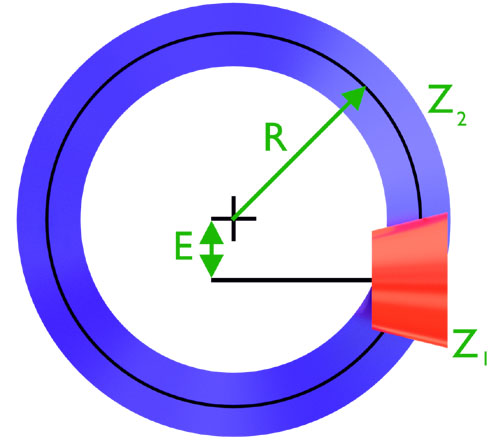Home  •  Tutorials  •  Calculators  •  3D Models  •  About  •  Contact

Hypoid Gear Calculator

Hypoid gears are similar to bevel gears but their shafts do not intersect. The following online calculator computes the basic dimensions, relative positions and tooth profiles of a hypoid gear pair (pinion and wheel) based on their number of teeth, the median radius of the wheel, and the hypoid shift. The angle between the shafts is assumed to be 90°.

A hypoid gear pair can be defined with as few as four parameters: the radius of the wheel's median circle (R), the hypoid shift (E) and the number of teeth on the pinion (z1) and wheel (z2). Strictly speaking, the reference surfaces for a hypoid pair are hyperboloids, but cones offer a reasonably good approximation.

This calculator takes R, E, z1 and z2 and computes the parametric equations for the tooth profiles, angular tooth thickness, transformation scripts, and tooth spiral angles for both gears. It also computes the position of the pinion relative to the wheel.Basic Dimensions
ParameterSymbolPinionWheel
Number of teethz
Hypoid ShiftE
Tooth thickness at base (°)ψb